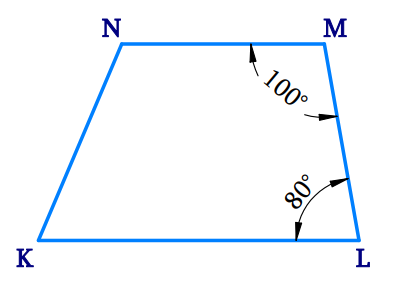# Ex.3.3 Q10 Understanding Quadrilaterals Solution-Ncert Maths Class 8

## Question

Explain how this figure is a trapezium. Which of its two sides are parallel?Video Solution
Understanding Quadrilaterals
Ex 3.3 | Question 10

## Text Solution

What is Known?

Given figure is a Quadrilateral.

What is Unknown?

To identify the two parallel sides of the figure and to prove that it is a trapezium.

Reasoning:

Trapezium is a quadrilateral having one pair of parallel sides.

Steps:

In the given figure $$KLMN$$,

$$\angle {\rm{L }}\,+$$ $${\rm{ }}\angle {\rm{M }}$$ $$= {\rm{18}}0^\circ$$ [two pair of adjacent angles (which form pairs of consecutive interior angles) are supplementary]

$= {\rm{ 8}}0^\circ {\rm{ }} + {\rm{1}}00^\circ = {\rm{18}}0^\circ$

Therefore, $$KN$$ is parallel to $$ML$$

Hence, $$KLMN$$ is a trapezium as it has a pair of parallel sides: $$KN$$ and $$ML$$.

Learn from the best math teachers and top your exams

• Live one on one classroom and doubt clearing
• Practice worksheets in and after class for conceptual clarity
• Personalized curriculum to keep up with school